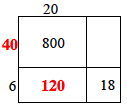### Home > CC1 > Chapter 6 > Lesson 6.1.1 > Problem6-9

6-9.

Consider the generic rectangle shown at right. Homework Help ✎

1. Copy and complete the generic rectangle.

Solve for the empty spaces one by one.
What is the product of $20$ and $6$?
Smaller steps like these make completing the whole rectangle easier.

A few of the spaces are filled in to help you get started.2. Write as many products as you can see in the rectangle. Find at least four. For each one, show the factors being multiplied, as well as the product.

One of these products is $20(40)$, which equals $800$.
Can you find at least three more like it?
If you are up for a challenge, there are eight more to find.

 $20$ $800$ $+6$ $18$